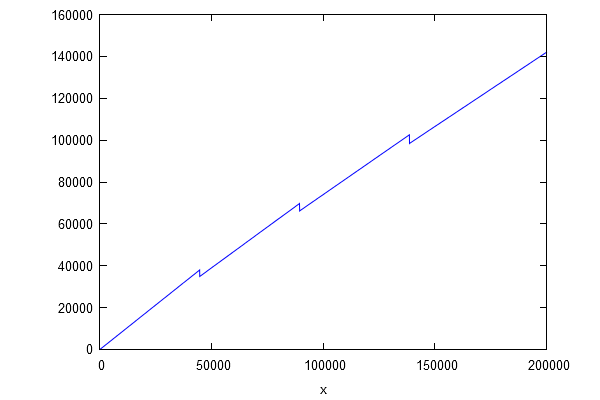## Wednesday, June 1, 2016

### Tax Brackets Are Not So Bad

There's a common misconception that many seem to have about the way taxes work. People overthink themselves about "moving into a higher tax bracket." They think that if they just barely go over their current tax bracket into the next, the tax man will get extra hungry and they will wind up making less money than if they, for example, didn't go in for that little bit of overtime. These concerns are, in a word, unwarranted. That's not to say that anecdotal evidence in support of this idea does not abound. I'm sure you can find anecdotal evidence for anything that's popular to complain about.

In 2015, the following tax brackets applied to income taxes:
• <=$44,701, 15% •$44,701—$89,401, 22% •$89,401—$138,586, 26% • >$138,586, 29%.
So, if I make $44,701 in taxable income, I pay$6705.15 in taxes on it. Suppose I make $44,702. Here's where the confusion becomes apparent. Some would try to tell you that you now pay 22% on the entire amount, or$9834.44, which would be a complaint worthy problem for someone teetering on the border of a tax bracket. (If a government actually tried to do it this way, the public outrage would produce headline news, not anecdotal evidence.) I call this false idea "folk" tax brackets.

What do I actually pay on \$44,702? I pay $$15\% \times 44,701 + 22\% \times 1 = 6705.37$$ in income tax.

The folk thinking taxation produces a jagged net income graph. This is the sort of thing people are implying when they speak of "making less" if they work overtime. You'd think they thought the take-home pay graph looked like this:Fig. 1. Marginal taxes don't cause the take-home pay graph to look anything like this, but some talk as if it did.
The real graph is not jagged. It isn't smooth either (if you look close enough). It is continuous, unlike the above graph, but it does produce corners at the locations of the tax bracket cross-overs. These are not really that scary looking:Fig. 2. Marginal taxes only increase the rate of tax "marginally". The increase in tax rate applies to the marginal amounts ("increments"), not the entire amounts. Basically you have a line with some very slight elbows in it where there is a small change in direction.
So, like you, I wouldn't mind if the whole graph was a little steeper (increased take-home pay percentage, less taxes), but it is what it is. And what it is is relatively fair, in concept at least.

### Modeling Taxes in Maxima

Now, where did I get these wonderful graphs? I produced them in Maxima, of course. Here's the Maxima code to define a marginal tax formula, if given the brackets that apply:

You can see the parts that you would need to change to produce your own formula for your marginal tax situation. FederalIncomeTax2015 is a list containing two lists. The first of these is the list of tax percentages. The next is the list of corresponding threshold values (0 is implicit as the beginning and the last percentage is assumed to apply to anything after the largest threshold amount).

Noteworthy items in the Maxima code above:

1. The characteristic function (charfun) produces the same effect as an if statement in the code. The characteristic function yields 1 if the condition is true, else 0. So, if an amount is not in the relevant range, it will not contribute. I have two different conditions to check for, but rather than branching, I just keep them in parallel. The advantage is simplicity. The disadvantage is that, in theory, there is more computation happening than really needs to happen. The simplicity carries a lot of weight in my book.
2. I append 0 and infinity to the list of boundaries for the tax brackets. Since Maxima recognizes inf and knows that any-number is less than inf, I have no case-taking to deal with the initial or final tax brackets.
3. map. When map will do the job, nothing does it more succinctly. Thanks map!
4. '' (quote-quote or double single-quote). Oh, boy. The basic meaning is, "evaluate what comes next and use the result of the evaluation instead of what's here." What this amounts to in this code is that the code that produces the function (the MarginalTaxFormula) is not re-run every time the function marginalFederalTax(income) is called with a different value of income. The MarginalTaxFormula(...) code is invoked by the '' operator and this returns the function which MarginalTaxFormual(...) produces. This is necessary because I am defining the function using the := operator which suspends evaluation until the function is invoked. Thus, you end up with a definition like this:
marginalFederalTax(income):=12788.1*charfun(income>=138586)+9834.0*charfun(income>=89401)+6705.15*charfun(income>=44701)+0.15*charfun(0
0.22*charfun(44701